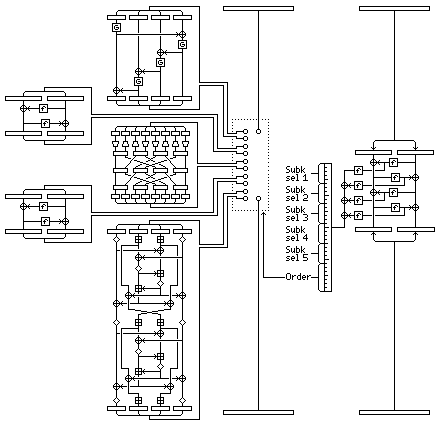[Next] [Up] [Previous] [Index]

# Mishmash

In thinking about ways to give a block cipher the kind of deep nonlinearity found in the rotor machine SIGABA, one idea I had is the kind of block cipher one round of which (for obvious reasons, I proposed only four rounds be used in it) is illustrated by the diagram below, and which I chose (also for reasons to become obvious) to call Mishmash:The cipher operates on a 128-bit block, and has a Feistel-like structure of sorts. The right half of the block is enciphered by four rounds of some block cipher with a 64-bit block and Feistel rounds, perhaps DES. The four f-function outputs of that process are also take, put through another f-function (otherwise, their XOR would be equivalent to the XOR of the two inputs and the two outputs), and XORed together to produce a 32-bit word that controls the encipherment of the left half of the block.

In a very schematic form, the left half of the diagram illustrates five operations to which the left half of the block will be subjected:

• Four rounds of Skipjack
• Two rounds of DES
• One round of SAFER
• Two rounds of some other cipher, perhaps Blowfish or QUADIBLOC, with Feistel rounds
• Two-round IDEA

Since IDEA is protected by patents, one will have to use both Blowfish and QUADIBLOC, or some other cipher instead for now; IDEA is shown because it has a unique basic structure.

Since 5! is 120, seven bits of the 32 bit word are sufficient to indicate the order in which the five operations are to be applied. 25 bits remain; 5 of them can be assigned to each of the 5 operations to choose one of 32 subkeys for each (there would be four separate sets of 32 subkeys for each for each of the four rounds of this block cipher as a whole).

[Next] [Up] [Previous] [Index]